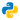# Intro to Webscraping

## Scrape a table from Wiki

This post covers these topics:

• Webscrape using BeautifulSoup.
• Use pandas to generate a table.
• Save the table in CSV and XLSX format.

We will be using this list below. The following list includes the largest South Korean companies by revenue in 2013 who are all listed as part of the Fortune Global 500.

``````import urllib2
from bs4 import BeautifulSoup
import pandas as pd

website = "https://en.wikipedia.org/wiki/South_Korea"

# Assign the html to the variable
my_html = urllib2.urlopen(website)

# Store the html in Beautiful Soup format
soup = BeautifulSoup(my_html, 'html.parser')

# Find and store the table in Beautiful Soup format
Table = soup.find('table', class_= 'wikitable sortable')

A = []
B = []
C = []
D = []
E = []

for row in Table.findAll('tr'):
cells = row.findAll('td')
if len(cells) > 1: # To extract table body only
A.append(cells.find(text=True))
B.append(cells.find(text=True))
C.append(cells.find(text=True))
D.append(cells.find(text=True))
E.append(cells.find(text=True))

# Create dataframe
df = pd.DataFrame()

df["Company Name"] = A
df["Revenue (mil)"] = C
df["Profit (mil)"] = D
df["Assets (mil)"] = E``````

Check the table `print df`. It should look something like this.

Now, let's create a CSV file.

``df.to_csv("seoul.csv", sep='\t') # Set delimeter to Tab``

To create an Excel file, first run this code in your terminal.

``pip install openpyxl``

After installing openpyxl, follow the instructions below.

``````writer = pd.ExcelWriter("seoul.xlsx")
df.to_excel(writer, 'Sheet1')
writer.save()``````

All done 😀